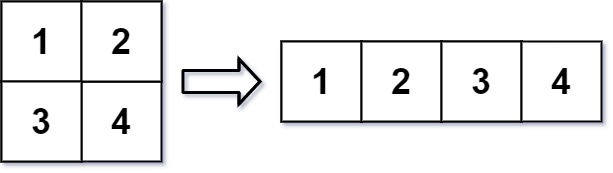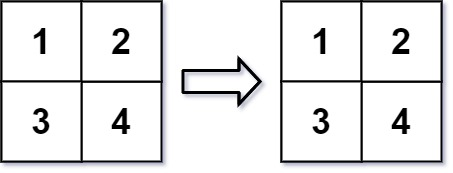# GeetCode Hub

In MATLAB, there is a handy function called `reshape` which can reshape an `m x n` matrix into a new one with a different size `r x c` keeping its original data.

You are given an `m x n` matrix `mat` and two integers `r` and `c` representing the row number and column number of the wanted reshaped matrix.

The reshaped matrix should be filled with all the elements of the original matrix in the same row-traversing order as they were.

If the `reshape` operation with given parameters is possible and legal, output the new reshaped matrix; Otherwise, output the original matrix.

Example 1:```Input: mat = [[1,2],[3,4]], r = 1, c = 4
Output: [[1,2,3,4]]
```

Example 2:```Input: mat = [[1,2],[3,4]], r = 2, c = 4
Output: [[1,2],[3,4]]
```

Constraints:

• `m == mat.length`
• `n == mat[i].length`
• `1 <= m, n <= 100`
• `-1000 <= mat[i][j] <= 1000`
• `1 <= r, c <= 300`

class Solution { public int[][] matrixReshape(int[][] mat, int r, int c) { } }